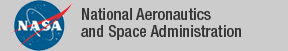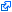### Field

Field is a set of PVSstrategies for simplifications and algebraic manipulations in the closed field of real numbers. The main strategy in the package is called `field` and it was originally based on a Coq tactic developed by Mayero and Delahaye. The strategy first removes the inverses that occur in a relational formula and then applies PVS decision procedures.

Field has been part of PVS since PVS 5.0. The current version of Field is 6.0 and is included in PVS 6.0. The strategies included in Field 6.0 are: `field`, `grind-reals`, `real-props`, `neg-formula`, `add-formulas`, `sub-formulas`, `cancel-by`, `cancel-formula`, `both-sides-f`, and `sq-simp`. This list is printed by the proof command `field-about`.

The tagidentifies links that are outside the NASA domain